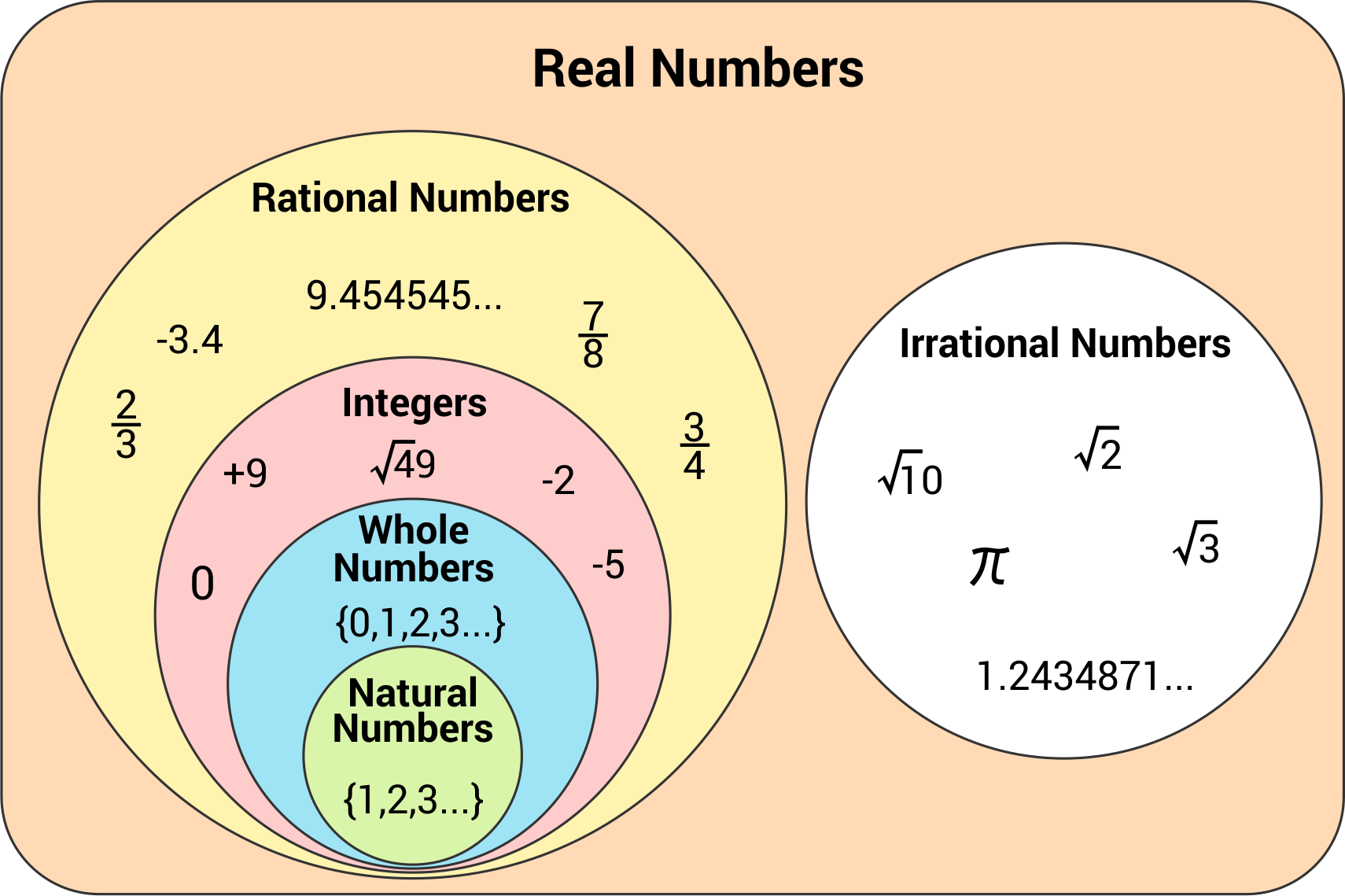# Integers, Decimals, and Fractions

Integers are whole numbers that are represented by tick marks on a number line. In between these marks, there are large spaces that are described as decimals and fractions. A fraction is a way to divide a section between integers into equally-spaced segments. A decimal representation divides the space between 0 and 1 into ten different segments.

### What is an integer?

#### A

An integer is any number, positive or negative, that does not have a fractional part. Examples of integers would be 5, 99, -4, 0, and 417.### What is a decimal?

#### A

“A decimal number is any number that uses a decimal point to show the part of the number that is less than 1. For example, the numbers 9.2, 16.327, and 0.4 are all decimal numbers. Pi is also a decimal number: 3.1415”

### Is 0 an integer?

#### A

Yes, 0 is an integer. An integer is any number that does not have a fractional part.

### Are all integers rational numbers?

#### A

Yes, all integers are rational numbers. A rational number is simply a number that can be found by dividing two integers.

### Are decimals integers?

#### A

No, decimals are not integers. According to the definition of an integer, they are numbers that do not have fractional parts. Ex. 6.25 = 6 $$\frac{1}{4}$$, which has a fractional part and is therefore not an integer

### Are whole numbers integers?

#### A

Yes, whole numbers are integers. A whole number is any number higher than zero that does not have a fractional component.

### Can integers be negative?

#### A

Yes, as long as there is no fractional component, integers can be negative.

747659688110

by Mometrix Test Preparation | This Page Last Updated: February 17, 2022

Get Actionable Study Tips
Join our newsletter to get the study tips, test-taking strategies, and key insights that high-performing students use.
Get Actionable Study Tips
Join our newsletter to get the study tips, test-taking strategies, and key insights that high-performing students use!